# Operators expressions-and-statements

6. Nov 2012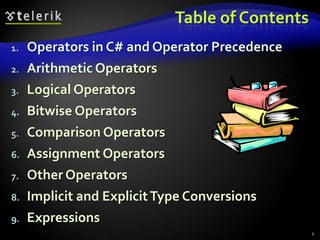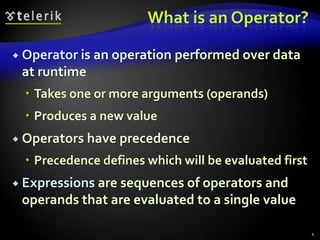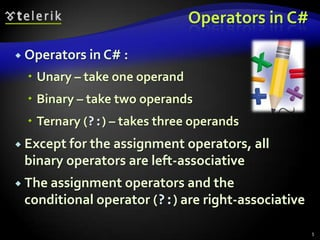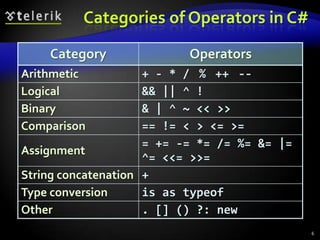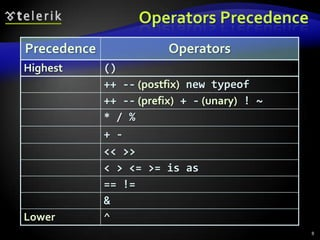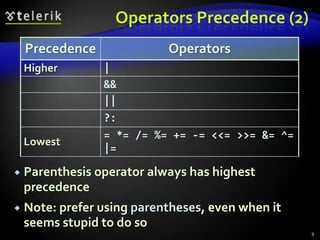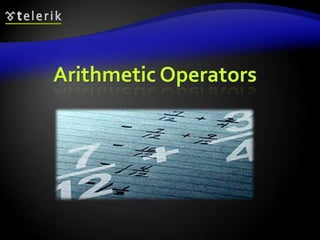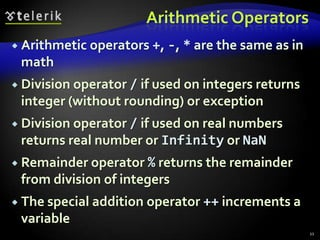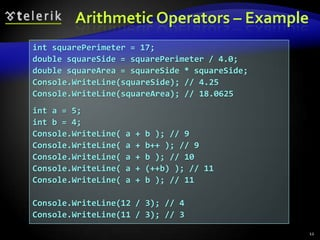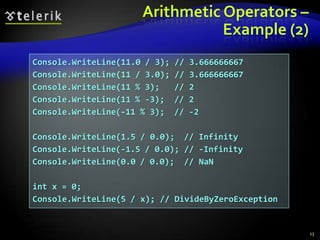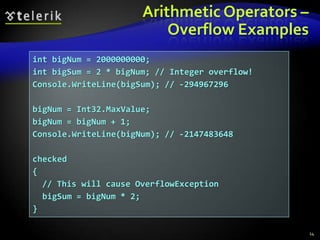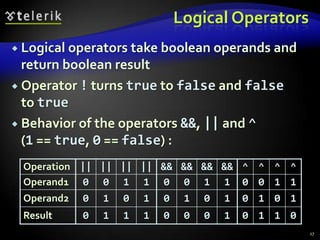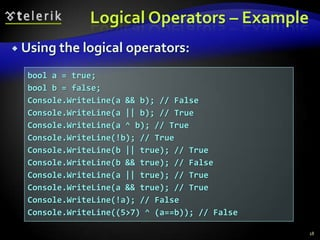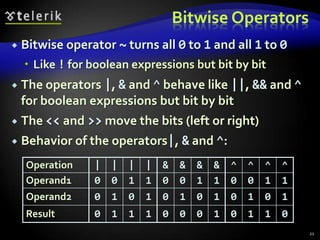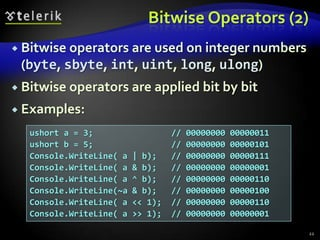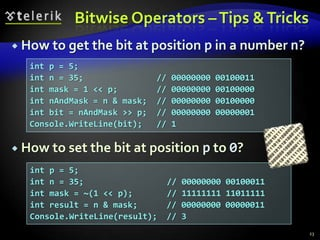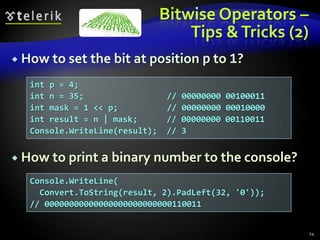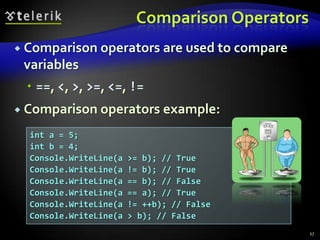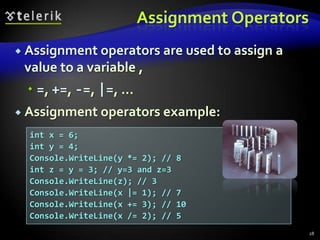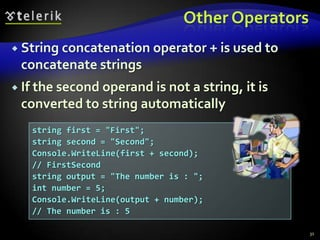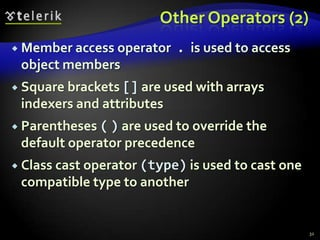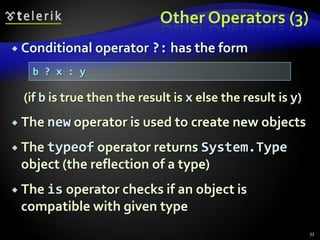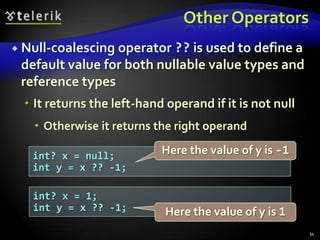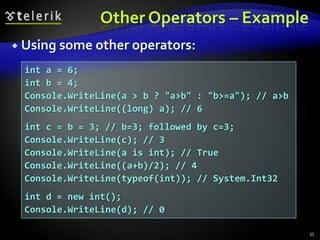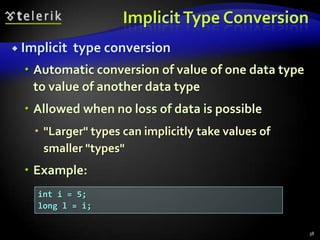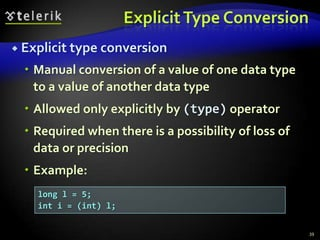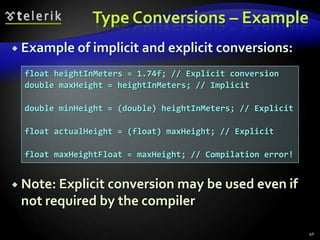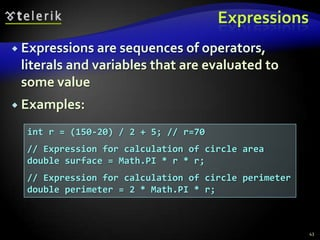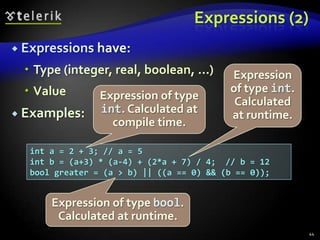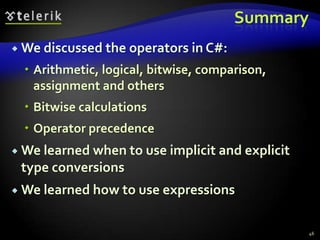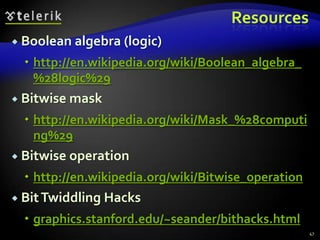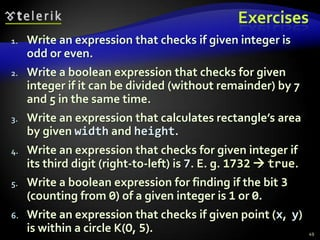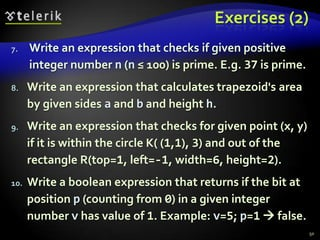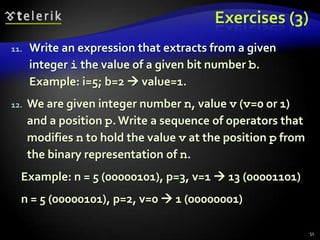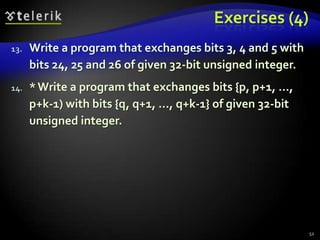1 von 52

### Operators expressions-and-statements

• 1. Operators and Expressions Performing Simple Calculations with C# Svetlin Nakov Telerik Corporation www.telerik.com
• 2. Table of Contents 1. Operators in C# and Operator Precedence 2. Arithmetic Operators 3. Logical Operators 4. Bitwise Operators 5. Comparison Operators 6. Assignment Operators 7. Other Operators 8. Implicit and Explicit Type Conversions 9. Expressions 2
• 3. Operators in C# Arithmetic, Logical, Comparison, Assignment, Etc.
• 4. What is an Operator?  Operator is an operation performed over data at runtime  Takes one or more arguments (operands)  Produces a new value  Operators have precedence  Precedence defines which will be evaluated first  Expressions are sequences of operators and operands that are evaluated to a single value 4
• 5. Operators in C#  Operators in C# :  Unary – take one operand  Binary – take two operands  Ternary (?:) – takes three operands  Except for the assignment operators, all binary operators are left-associative  The assignment operators and the conditional operator (?:) are right-associative 5
• 6. Categories of Operators in C# Category Operators Arithmetic + - * / % ++ -- Logical && || ^ ! Binary & | ^ ~ << >> Comparison == != < > <= >= = += -= *= /= %= &= |= Assignment ^= <<= >>= String concatenation + Type conversion is as typeof Other . [] () ?: new 6
• 8. Operators Precedence Precedence Operators Highest () ++ -- (postfix) new typeof ++ -- (prefix) + - (unary) ! ~ * / % + - << >> < > <= >= is as == != & Lower ^ 8
• 9. Operators Precedence (2) Precedence Operators Higher | && || ?: = *= /= %= += -= <<= >>= &= ^= Lowest |=  Parenthesis operator always has highest precedence  Note: prefer using parentheses, even when it seems stupid to do so 9
• 11. Arithmetic Operators  Arithmetic operators +, -, * are the same as in math  Division operator/ if used on integers returns integer (without rounding) or exception  Division operator / if used on real numbers returns real number or Infinity or NaN  Remainder operator % returns the remainder from division of integers  The special addition operator ++ increments a variable 11
• 12. Arithmetic Operators – Example int squarePerimeter = 17; double squareSide = squarePerimeter / 4.0; double squareArea = squareSide * squareSide; Console.WriteLine(squareSide); // 4.25 Console.WriteLine(squareArea); // 18.0625 int a = 5; int b = 4; Console.WriteLine( a + b ); // 9 Console.WriteLine( a + b++ ); // 9 Console.WriteLine( a + b ); // 10 Console.WriteLine( a + (++b) ); // 11 Console.WriteLine( a + b ); // 11 Console.WriteLine(12 / 3); // 4 Console.WriteLine(11 / 3); // 3 12
• 13. Arithmetic Operators – Example (2) Console.WriteLine(11.0 / 3); // 3.666666667 Console.WriteLine(11 / 3.0); // 3.666666667 Console.WriteLine(11 % 3); // 2 Console.WriteLine(11 % -3); // 2 Console.WriteLine(-11 % 3); // -2 Console.WriteLine(1.5 / 0.0); // Infinity Console.WriteLine(-1.5 / 0.0); // -Infinity Console.WriteLine(0.0 / 0.0); // NaN int x = 0; Console.WriteLine(5 / x); // DivideByZeroException 13
• 14. Arithmetic Operators – Overflow Examples int bigNum = 2000000000; int bigSum = 2 * bigNum; // Integer overflow! Console.WriteLine(bigSum); // -294967296 bigNum = Int32.MaxValue; bigNum = bigNum + 1; Console.WriteLine(bigNum); // -2147483648 checked { // This will cause OverflowException bigSum = bigNum * 2; } 14
• 15. Arithmetic Operators Live Demo
• 17. Logical Operators  Logical operators take boolean operands and return boolean result  Operator ! turns true to false and false to true  Behavior of the operators &&, || and ^ (1 == true, 0 == false) : Operation || || || || && && && && ^ ^ ^ ^ Operand1 0 0 1 1 0 0 1 1 0 0 1 1 Operand2 0 1 0 1 0 1 0 1 0 1 0 1 Result 0 1 1 1 0 0 0 1 0 1 1 0 17
• 18. Logical Operators – Example  Using the logical operators: bool a = true; bool b = false; Console.WriteLine(a && b); // False Console.WriteLine(a || b); // True Console.WriteLine(a ^ b); // True Console.WriteLine(!b); // True Console.WriteLine(b || true); // True Console.WriteLine(b && true); // False Console.WriteLine(a || true); // True Console.WriteLine(a && true); // True Console.WriteLine(!a); // False Console.WriteLine((5>7) ^ (a==b)); // False 18
• 19. Logical Operators Live Demo
• 21. Bitwise Operators  Bitwise operator ~ turns all 0 to 1 and all 1 to 0  Like ! for boolean expressions but bit by bit  The operators |, & and ^ behave like ||, && and ^ for boolean expressions but bit by bit  The << and >> move the bits (left or right)  Behavior of the operators|, & and ^: Operation | | | | & & & & ^ ^ ^ ^ Operand1 0 0 1 1 0 0 1 1 0 0 1 1 Operand2 0 1 0 1 0 1 0 1 0 1 0 1 Result 0 1 1 1 0 0 0 1 0 1 1 0 21
• 22. Bitwise Operators (2)  Bitwise operators are used on integer numbers (byte, sbyte, int, uint, long, ulong)  Bitwise operators are applied bit by bit  Examples: ushort a = 3; // 00000000 00000011 ushort b = 5; // 00000000 00000101 Console.WriteLine( a | b); // 00000000 00000111 Console.WriteLine( a & b); // 00000000 00000001 Console.WriteLine( a ^ b); // 00000000 00000110 Console.WriteLine(~a & b); // 00000000 00000100 Console.WriteLine( a << 1); // 00000000 00000110 Console.WriteLine( a >> 1); // 00000000 00000001 22
• 23. Bitwise Operators – Tips & Tricks  How to get the bit at position p in a number n? int p = 5; int n = 35; // 00000000 00100011 int mask = 1 << p; // 00000000 00100000 int nAndMask = n & mask; // 00000000 00100000 int bit = nAndMask >> p; // 00000000 00000001 Console.WriteLine(bit); // 1  How to set the bit at position p to 0? int p = 5; int n = 35; // 00000000 00100011 int mask = ~(1 << p); // 11111111 11011111 int result = n & mask; // 00000000 00000011 Console.WriteLine(result); // 3 23
• 24. Bitwise Operators – Tips & Tricks (2)  How to set the bit at position p to 1? int p = 4; int n = 35; // 00000000 00100011 int mask = 1 << p; // 00000000 00010000 int result = n | mask; // 00000000 00110011 Console.WriteLine(result); // 3  How to print a binary number to the console? Console.WriteLine( Convert.ToString(result, 2).PadLeft(32, '0')); // 00000000000000000000000000110011 24
• 25. Bitwise Operators Live Demo
• 27. Comparison Operators  Comparison operators are used to compare variables  ==, <, >, >=, <=, !=  Comparison operators example: int a = 5; int b = 4; Console.WriteLine(a >= b); // True Console.WriteLine(a != b); // True Console.WriteLine(a == b); // False Console.WriteLine(a == a); // True Console.WriteLine(a != ++b); // False Console.WriteLine(a > b); // False 27
• 28. Assignment Operators  Assignment operators are used to assign a value to a variable ,  =, +=, -=, |=, ...  Assignment operators example: int x = 6; int y = 4; Console.WriteLine(y *= 2); // 8 int z = y = 3; // y=3 and z=3 Console.WriteLine(z); // 3 Console.WriteLine(x |= 1); // 7 Console.WriteLine(x += 3); // 10 Console.WriteLine(x /= 2); // 5 28
• 31. Other Operators  String concatenation operator + is used to concatenate strings  If the second operand is not a string, it is converted to string automatically string first = "First"; string second = "Second"; Console.WriteLine(first + second); // FirstSecond string output = "The number is : "; int number = 5; Console.WriteLine(output + number); // The number is : 5 31
• 32. Other Operators (2)  Member access operator . is used to access object members  Square brackets [] are used with arrays indexers and attributes  Parentheses ( ) are used to override the default operator precedence  Class cast operator (type) is used to cast one compatible type to another 32
• 33. Other Operators (3)  Conditional operator ?: has the form b ? x : y (if b is true then the result is x else the result is y)  The new operator is used to create new objects  The typeof operator returns System.Type object (the reflection of a type)  The is operator checks if an object is compatible with given type 33
• 34. Other Operators  Null-coalescing operator ?? isused to define a default value for both nullable value types and reference types  It returns the left-hand operand if it is not null  Otherwise it returns the right operand int? x = null; Here the value of y is -1 int y = x ?? -1; int? x = 1; int y = x ?? -1; Here the value of y is 1 34
• 35. Other Operators – Example  Using some other operators: int a = 6; int b = 4; Console.WriteLine(a > b ? "a>b" : "b>=a"); // a>b Console.WriteLine((long) a); // 6 int c = b = 3; // b=3; followed by c=3; Console.WriteLine(c); // 3 Console.WriteLine(a is int); // True Console.WriteLine((a+b)/2); // 4 Console.WriteLine(typeof(int)); // System.Int32 int d = new int(); Console.WriteLine(d); // 0 35
• 36. Other Operators Live Demo
• 37. Implicit and Explicit Type Conversions
• 38. Implicit Type Conversion  Implicit type conversion  Automatic conversion of value of one data type to value of another data type  Allowed when no loss of data is possible  "Larger" types can implicitly take values of smaller "types"  Example: int i = 5; long l = i; 38
• 39. Explicit Type Conversion  Explicit type conversion  Manual conversion of a value of one data type to a value of another data type  Allowed only explicitly by (type) operator  Required when there is a possibility of loss of data or precision  Example: long l = 5; int i = (int) l; 39
• 40. Type Conversions – Example  Example of implicit and explicit conversions: float heightInMeters = 1.74f; // Explicit conversion double maxHeight = heightInMeters; // Implicit double minHeight = (double) heightInMeters; // Explicit float actualHeight = (float) maxHeight; // Explicit float maxHeightFloat = maxHeight; // Compilation error!  Note: Explicit conversion may be used even if not required by the compiler 40
• 41. Type Conversions Live Demo
• 43. Expressions  Expressions are sequences of operators, literals and variables that are evaluated to some value  Examples: int r = (150-20) / 2 + 5; // r=70 // Expression for calculation of circle area double surface = Math.PI * r * r; // Expression for calculation of circle perimeter double perimeter = 2 * Math.PI * r; 43
• 44. Expressions (2)  Expressions have:  Type (integer, real, boolean, ...) Expression  Value of type int. Expression of type Calculated  Examples: int. Calculated at at runtime. compile time. int a = 2 + 3; // a = 5 int b = (a+3) * (a-4) + (2*a + 7) / 4; // b = 12 bool greater = (a > b) || ((a == 0) && (b == 0)); Expression of type bool. Calculated at runtime. 44
• 46. Summary  We discussed the operators in C#:  Arithmetic, logical, bitwise, comparison, assignment and others  Bitwise calculations  Operator precedence  We learned when to use implicit and explicit type conversions  We learned how to use expressions 46
• 47. Resources  Boolean algebra (logic)  http://en.wikipedia.org/wiki/Boolean_algebra_ %28logic%29  Bitwise mask  http://en.wikipedia.org/wiki/Mask_%28computi ng%29  Bitwise operation  http://en.wikipedia.org/wiki/Bitwise_operation  Bit Twiddling Hacks  graphics.stanford.edu/~seander/bithacks.html 47
• 48. Operators and Expressions Questions? http://academy.telerik.com
• 49. Exercises 1. Write an expression that checks if given integer is odd or even. 2. Write a boolean expression that checks for given integer if it can be divided (without remainder) by 7 and 5 in the same time. 3. Write an expression that calculates rectangle’s area by given width and height. 4. Write an expression that checks for given integer if its third digit (right-to-left) is 7. E. g. 1732  true. 5. Write a boolean expression for finding if the bit 3 (counting from 0) of a given integer is 1 or 0. 6. Write an expression that checks if given point (x, y) is within a circle K(O, 5). 49
• 50. Exercises (2) 7. Write an expression that checks if given positive integer number n (n ≤ 100) is prime. E.g. 37 is prime. 8. Write an expression that calculates trapezoid's area by given sides a and b and height h. 9. Write an expression that checks for given point (x, y) if it is within the circle K( (1,1), 3) and out of the rectangle R(top=1, left=-1, width=6, height=2). 10. Write a boolean expression that returns if the bit at position p (counting from 0) in a given integer number v has value of 1. Example: v=5; p=1  false. 50
• 51. Exercises (3) 11. Write an expression that extracts from a given integer i the value of a given bit number b. Example: i=5; b=2  value=1. 12. We are given integer number n, value v (v=0 or 1) and a position p. Write a sequence of operators that modifies n to hold the value v at the position p from the binary representation of n. Example: n = 5 (00000101), p=3, v=1  13 (00001101) n = 5 (00000101), p=2, v=0  1 (00000001) 51
• 52. Exercises (4) 13. Write a program that exchanges bits 3, 4 and 5 with bits 24, 25 and 26 of given 32-bit unsigned integer. 14. * Write a program that exchanges bits {p, p+1, …, p+k-1) with bits {q, q+1, …, q+k-1} of given 32-bit unsigned integer. 52